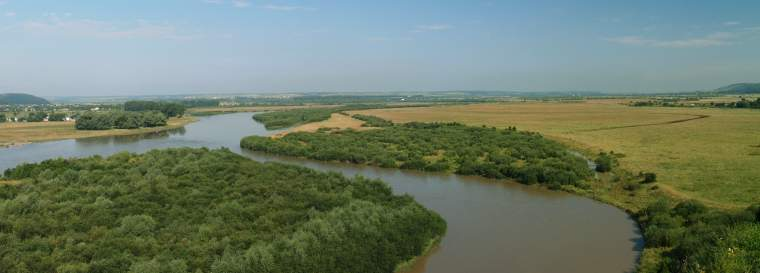﻿ Yuri A.W. Shardt: Research## Data Quality Assessment

Data quality assessment is a burgeoning field in the area of data mining for applications in system identification . As the amount of data stored in industrial data historians increases exponentially, its usefulness is harder to manually determine. Manually parsing large data sets consisting of fifty or more variables sampled every minute for one or two years is practically impossible. Furthermore, it may be useful to automatically extract valuable data regions from a given data set for online process modelling, for example, in just-in-time modelling. Data quality assessment finds applications in many different fields including soft-sensor development [1, 6], process optimisation , control , and process monitoring. Figure 1 shows the general data quality assessment procedure.

The data quality assessment procedure can be summarised as [3, 7, 6]:

1. Preprocessing: In this step, the data set is loaded and analysed. Often, the data set will be mean scaled and centred. As well, known thresholds and limits will be considered at this point.
2. Mode changes: It may be known how the operating modes of the process change, for example, the controller modes could change based on the relationships between rt and ut. As well, it may be the case that the specific mode will determine how much data is required to obtain good parameter estimates.
3. Partitioning: For each identified mode, perform the following steps:
1. Initialisation: If the length of the unanalysed data for the given mode is greater than the minimum required length r, set the model counter to the current data point, kinit = k and then set k = k + r. Otherwise, go to the next identified mode.
2. Preprocessing: For certain types of processes, it may be necessary to perform additional manipulations, for example, for an integrating process, it is necessary to integrate the input.
3. Computation: Compute the required values. In most cases, this will include the variances of the signals and the condition number of the information matrix.
4. Comparison: Compare the variances, the condition number of the regressor matrix, and the significance of the parameters against the thresholds.
1. Failure: If any of the thresholds fail to be met go to the next data point, that is, k = k + 1, and go to Step 3.1.
2. Success: Otherwise, set k = k + 1, and go to Step 3.2. The “good” data region is then [kinit, k].
3. Termination: The procedure stops once k equals N, the total number of data points in the given mode. Repeat Step 3 for any remaining modes.
4. Simplification: To improve performance and obtain longer data sets, adjacent regions can be compared and determined if they could be considered to come from a single model.
It should be noted that in practice, the required variances are computed using a recursive method that involves the tuning of additional forgetting factors. In practice, setting the tunable parameters and the various thresholds can be difficult since the signal properties can vary greatly and it can be difficult to set general conditions that can cover most, if not all cases.

### Resources

The following digital resources are available for data quality assessment:

• GitHub repository for the raw MATLAB code: https://github.com/Yuri-Research/DQ